## Basics behind BJT and FET experiments in PSLab

A high school student in his curriculum; will come across certain electronics and electrical experiments. One of them related to semiconductor devices such as Bipolar Junction Transistors (BJTs) and Field Effect Transistors (FETs). PSLab device is capable of function as a waveform generator, voltage and current source, oscilloscope and multimeter. Using these functionalities one can design an experiment. This blog post brings out the basics one should know about the experiment and the PSLab device to program an experiment in the saved experiments section.

Channels and Sources in the PSLab Device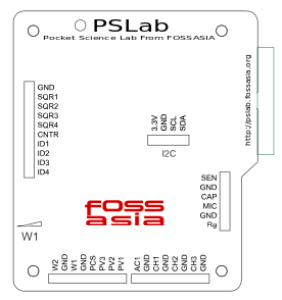The PSLab device has three pins dedicated to function as programmable voltage sources (PVS) and one pin for programmable current source (PCS).

Programmable Voltage Sources can generate voltages as follows;

• PV1 →  -5V ~ +5V
• PV2 → -3.3V ~ +3.3V
• PV3 → 0 ~ +3.3V

Programmable Current Source (PCS) can generate current as follows;

• PCS → 0 ~ 3.3mA

The device has 4 channel oscilloscope out of those CH1, CH2 and CH3 pins are useful in experiments of the current context type.

Every semiconductor device is made of Silicon(Si). Some are made of Germanium(Ge) but they are not widely used. Silicon material has a potential barrier of 0.7 V among P type and N type sections of a semiconductor device. This voltage value is really important in an experiment as in some practicals such as “BJT Amplifier”, there is no use of a voltage value setting below this value. So the experiment needs to be programmed to have 0.7V as the minimum voltage for Base terminal.

Basic BJT experiments

BJTs have three pins. Collector, Emitter and Base. Current to the Base pin will control the flow of electrons from Emitter to Collector creating a voltage difference between Collector and Emitter pins. This scenario can be taken down to three types as;

• Input Characteristics → Relationship between Emitter current to VBE(Base to Emitter)
• Output Characteristics → Relationship between IC(Collector) to VCB(Collector to Base)
• Transfer Characteristics → Relationship between IC(Collector) to IE(Emitter)
 Input Characteristics Output Characteristics Transfer Characteristics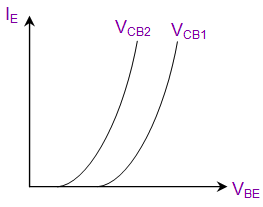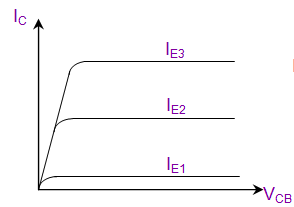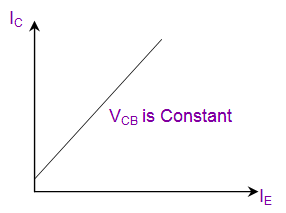Basic FET experiments

FETs have three pins. Drain, Source and Gate. Voltage to Gate terminal will control the electron flow from either direction from or to Source and Drain. This scenario results in two types of experiments;

• Output Characteristics → Drain current to Drain to Source voltage difference
• Transfer Characteristics → Gate to Source voltage to Drain current
 Output Characteristics Transfer Characteristics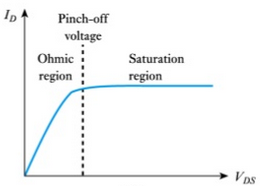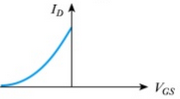Using existing methods in PSLab android repository

Current implementation of the android application consists of all the methods required to read voltages and currents from the relevant pins and fetch waveforms from the channel pins and output voltages from PVS pins.

ScienceLab.java class – This class implements all the methods required for any kind of an experiment. The methods that will be useful in designing BJT and FET related experiments are;

Set Voltages

```public void setPV1(float value);
```

```public void setPV2(float value);
```

```public void setPV3(float value);
```

Set Currents

```public void setPCS(float value);
```

```public double getVoltage(String channelName, Integer sample);
```

To read current there is no direct way implemented. The current flow between two nodes can be calculated using the PVS pin value and the voltage value read from the channel pins. It uses Ohm’s law to calculate the value using the known resistance between two nodes.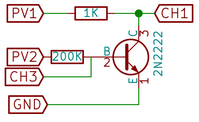In the following schematic; the collector current can be calculated using known PV1 value and the measured CH1 value as follows;

IC = (PV1 – CH1) / 1000

This is how it is actually implemented in the existing experiments.

If one needs to implement a new experiment of any kind, these are the basics need to know. There can be so many new experiments implemented using these basics. Some of them could be;

• Effect of Temperature coefficient in Collector current
• The influence in β factor in Collector current

Resources:

## Implementing Experiment Functionality in PSLab Android

Using the PSLab Hardware Device, users can perform experiments in various domains like Electronics, Electrical, Physics, School Level experiments, etc. These experiments can be performed using functionalities exposed by hardware device like Programmable Voltage Sources, Programmable Current Source, etc. In this post we will try implementing the functionality to perform an experiment using the PSLab Hardware Device and the PSLab Android App.

Let us take the Ohm’s law experiment as an example and see how it’s implement using the  PSLab Android App.

Ohm’s law states that the current through a conductor between two points is directly proportional to the voltage across the two points, effectively using a constant of proportionality called Resistance (R) where,

`R = V / I`

#### Schematic#### Layout to perform Ohm’s law experiment

The Ohm’s law experiment requires a variable current, so a seekbar is provided to change the current coming from PCS channel, values of which are continuously reflected in the TextView next to it.#### Implementation

The Read button has a listener attached to it. Once it is clicked, the currentValue is updated with the value parsed from the seekbar progress and the selectedChannel variable is assigned from the spinner. These variables are used by the background thread to change the current supplied by current source (PCS pin) of the device and to read the updated voltage from the selected channel of the device.

```btnReadVoltage.setOnClickListener(new View.OnClickListener() {
@Override
public void onClick(View v) {
selectedChannel = channelSelectSpinner.getSelectedItem().toString();
currentValue = Double.parseDouble(tvCurrentValue.getText().toString());
if (scienceLab.isConnected()) {
CalcDataPoint calcDataPoint = new CalcDataPoint();
calcDataPoint.execute();
} else {
Toast.makeText(getContext(), "Device not connected", Toast.LENGTH_SHORT).show();
}
}
});```

CalcDataPoint is an AsyncTask which does all the underlying work like setting the current at the PCS channel, reading the voltage from the CH1 channel and triggering the update of the data points on the graph.

```private class CalcDataPoint extends AsyncTask<Void, Void, Void> {

@Override
protected Void doInBackground(Void... params) {
scienceLab.setPCS((float) currentValue);
switch (selectedChannel) {
case "CH1":
voltageValue = scienceLab.getVoltage("CH1", 5);
break;
case "CH2":
voltageValue = scienceLab.getVoltage("CH2", 5);
break;
case "CH3":
voltageValue = scienceLab.getVoltage("CH3", 5);
break;
default:
voltageValue = scienceLab.getVoltage("CH1", 5);
}
return null;
}

@Override
protected void onPostExecute(Void aVoid) {
super.onPostExecute(aVoid);
updateGraph();
}
}```

updateGraph() method is used to update the graph on UI thread. It creates a new dataset from the points which were added by the background thread and refreshes the graph with it using the invalidate() method.

```private void updateGraph() {
tvVoltageValue.setText(df.format(voltageValue));
List<ILineDataSet> dataSets = new ArrayList<>();
List<Entry> temp = new ArrayList<>();
for (int i = 0; i < x.size(); i++) {
}
LineDataSet dataSet = new LineDataSet(temp, "I-V Characteristic");
dataSet.setColor(Color.RED);
dataSet.setDrawValues(false);